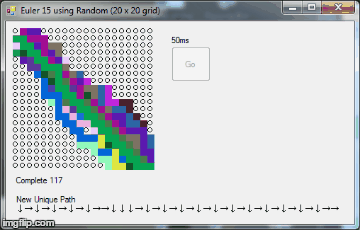# Chicago Gun Shootings 2015

A different take on Chicago Tribune’s Gun Shooting visualizations. Time Range: JAN. 1, 2015 through AUG. 31, 2015.

The data was obtained and parsed from the Trib http://crime.chicagotribune.com/chicago/shootings

I made a daily line chart using Google Charts, and an overall intensity map using CartoDB.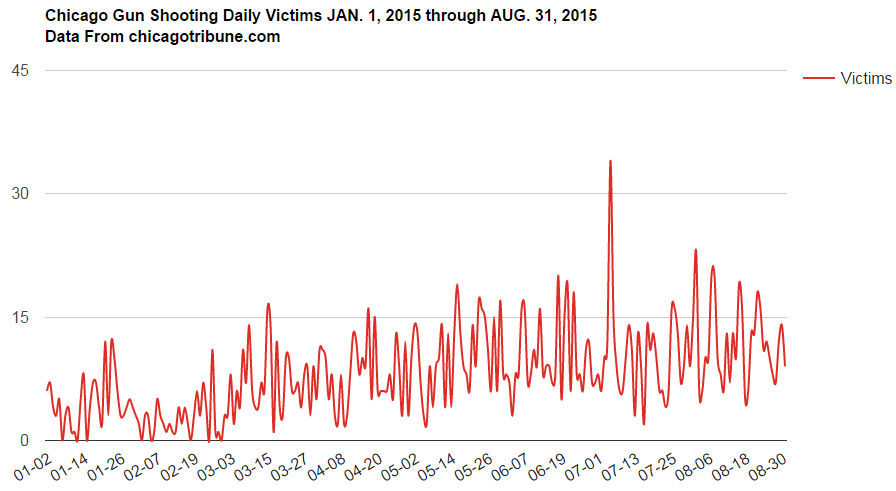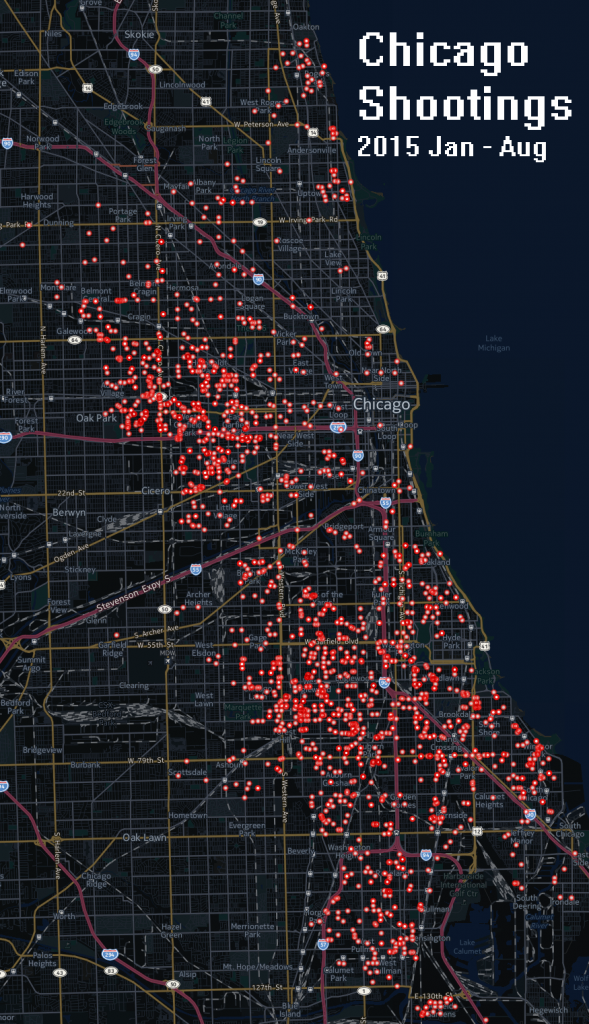# Comcast’s Monetary Influence Over Illinois Political Candidates

I found an interesting dataset on political contributions in the state of Illinois.. The downloadable .zip contains multiple tab delimited database files which contain the relationships between Donations, Committees, and Candidates.

Out of curiosity in seeing Comcast’s political influence in Illinois over time, I parsed the 650,775mb file called Receipts.txt. Below is a bar chart of yearly recorded donation totals from 2000 through 2015-08.

Not being very politically oriented, I wanted to somehow relate the donations to candidates. But in the form of the available data, it appears Donors make contributions to Committees, and Committees support a Candidate. But I do not know if Candidates and Committees are a One to One relationship at the time of typing this.

Parsing the text file called CmteCandidateLinks.txt, I related the Committee Id with the candidate Id. Parsing the text file called Candidates.txt I relate the Candidate Id to the Candidate name.

Lots of candidate duplicates per donation entry. Majority of Committees represent the same candidate under different ids, while some committees represent multiple candidates. Example here: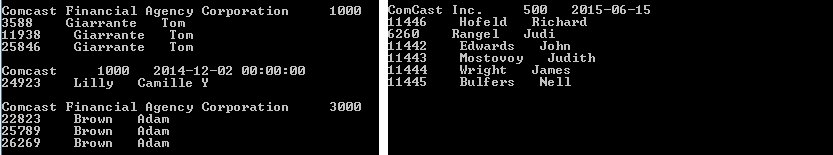So I decided to distribute each donation amount between a potential multitude of candidates. I did this by dividing each donation by the number of candidates which belong to the committee recipient. From that, I got this list of Comcast’s Top Illinois Candidates.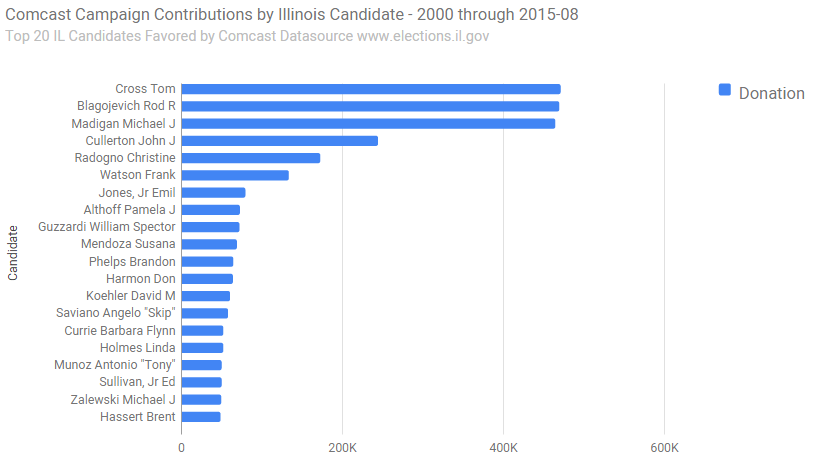# Vietnam conflict charts and graphs

In this post I will be exploring some data I found about the Vietnam War.
The data is from The National Archives Catalog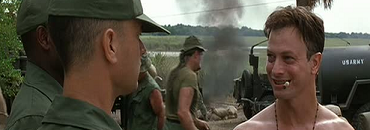The downloaded data file DCAS.VN.EXT08.DAT contains 58,220 records. Each row appears to be an individual involved in the war. I couldn’t make immediate sense of the cookbook documents, so I proceeded straight to parsing it raw. By eyeballing the values of each column I was able to determine the following attributes per row:

Name, Branch, Rank, Assigned Position, Gender, Hometown, Country, State, Relationship Status, Religion, Race, Mortility Status, and Reason of Death.

C# Console:

```Console.BufferHeight = 4000; Console.WriteLine("Charlie in the Trees");   System.IO.StreamReader myFile = new System.IO.StreamReader(@"C:\VIETNAM\DCAS.VN.EXT08.DAT"); string vietnamData = myFile.ReadToEnd(); myFile.Close(); string[] lines = vietnamData.Split(new string[] { Environment.NewLine }, StringSplitOptions.None); int count = 0; foreach( string line in lines ) { string[] parts = line.Split('|'); Console.WriteLine("name : " + parts); Console.WriteLine("branch : " + parts); Console.WriteLine("rank : " + parts); /* ... */ Console.WriteLine(); count += 1; } Console.WriteLine(); Console.WriteLine(); Console.WriteLine("total " + count);```

output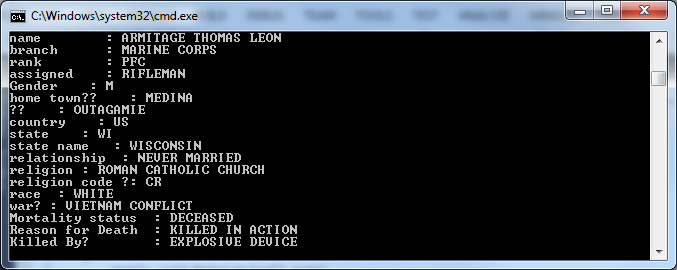Alright, now that we’ve parsed the data, I typically ask myself these questions:
what is interesting?
what do I want to see?
what might be controversial?
what could invoke the attention of others?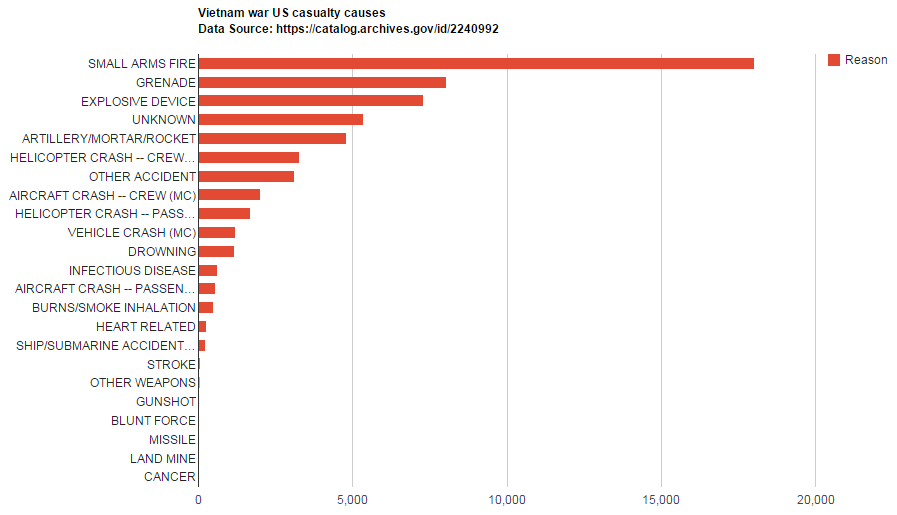```Dictionary<string, int> dictConcepts = new Dictionary<string, int>(); foreach( string line in lines ) { string[] parts = line.Split('|'); if (parts == "DECEASED") { if (dictConcepts.ContainsKey(parts)) { int cur_count = dictConcepts[parts]; cur_count += 1; dictConcepts[parts] = cur_count; } else { dictConcepts.Add(parts, 1); } } }```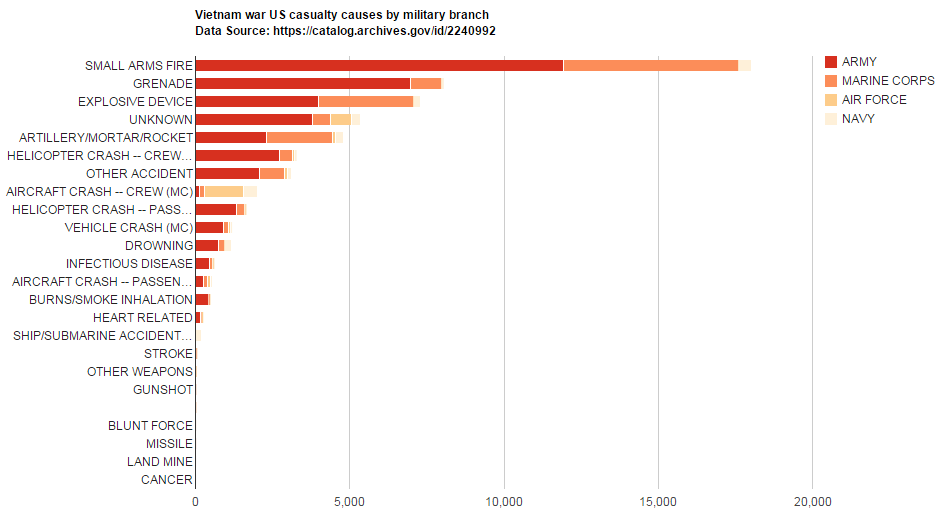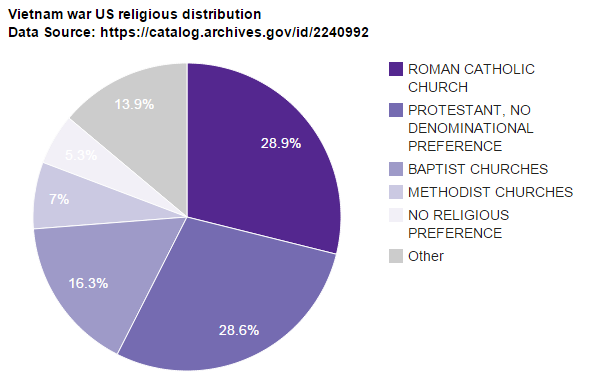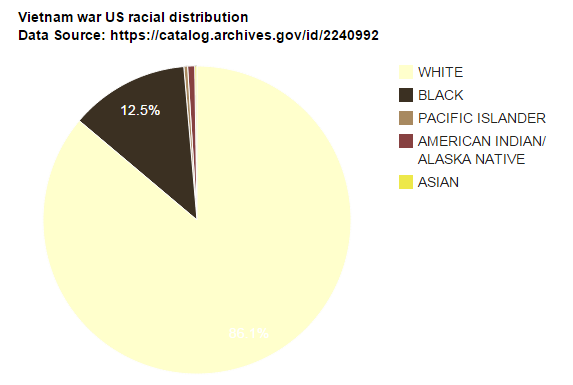# Words used in Tumblr’s usernames (380,000 users)

C# was written to:

• parse an English dictionary for words. Link here
• search for each English word with a length of 5 or greater within each username
• store each English word found, and count its frequency throughout all names
• Wordle was used to generate a word cloud of the most frequent words.

Most Frequent English Words Found in TUMBLR’s Usernames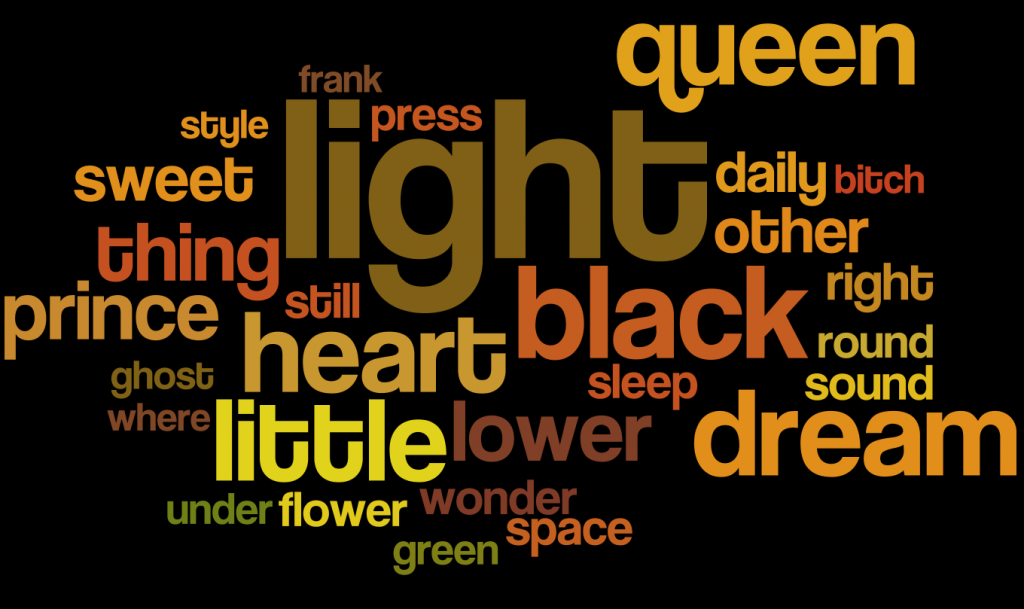# C#: Tumblr Blog Description Top Word Frequency List Visualization

Browsing reddit; I found a parsed dataset from Tublr. Link Here.
I used code from an older post to obtain a list of unique words, and their frequencies.
Next, I used a default TermCloud – Sample from GoogleCharts’s Additional Charts Gallery, to generate this image in a web browser.

Tublr blog description: words with a frequency greater than 5,000, ordered by most to least frequent.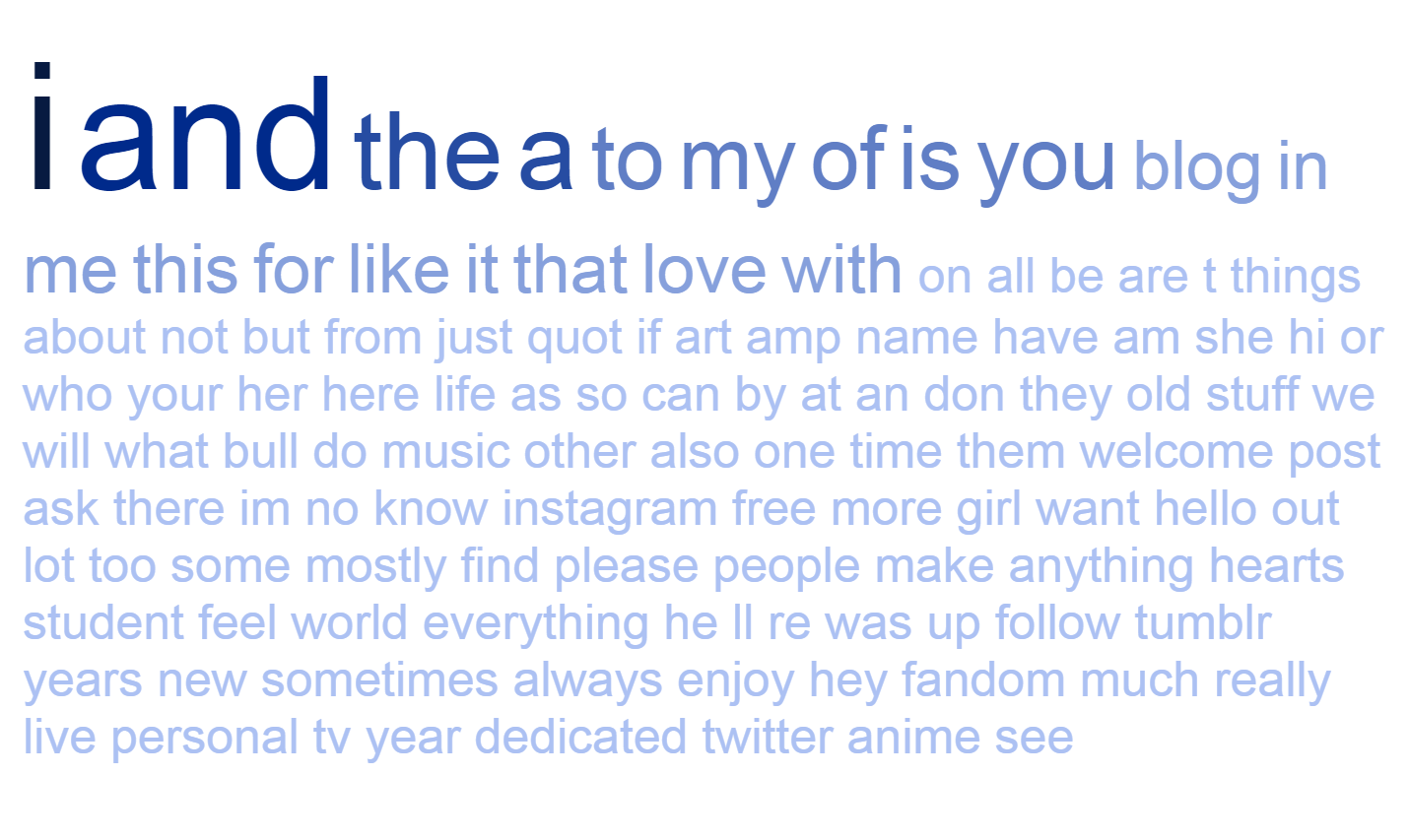```using System; using System.Collections.Generic; using System.Linq; using System.Text; using System.IO;   namespace ordertumblrwords { class Program { static void Main(string[] args) { int counter = 0; string line; Dictionary<string, int> hashfreq = new Dictionary<string, int>(); System.IO.StreamReader file = new System.IO.StreamReader("C:\\Book\\New folder\\OUTPUT.txt"); while ((line = file.ReadLine()) != null) { string[] parts = line.Split(':'); int freq = int.Parse(parts.ToString().Trim()); hashfreq.Add(parts, freq); counter++; } file.Close(); int q = 0; string masterstring = ""; foreach (KeyValuePair<string, int> item in hashfreq.OrderByDescending(key => key.Value)) { if (item.Value > 5000) { Console.WriteLine("data.setValue(" + q + ", 0, '" + item.Key + "');"); Console.WriteLine("data.setValue(" + q + ", 1, "+item.Value+");"); q += 1; masterstring += "data.setValue(" + q + ", 0, '" + item.Key + "');\r\n"; masterstring += "data.setValue(" + q + ", 1, " + item.Value + ");\r\n"; }   } StreamWriter streamWrite; streamWrite = File.AppendText("C:\\Book\\MANTASMAIN.txt"); streamWrite.WriteLine(masterstring); streamWrite.Close(); } } }```

# C#: Euler 46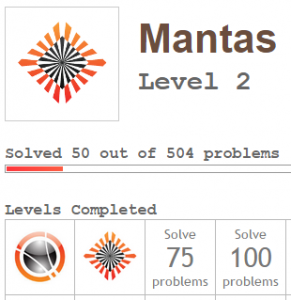Goldbach’s other conjecture

https://projecteuler.net/problem=46
It was proposed by Christian Goldbach that every odd composite number can be written as the sum of a prime and twice a square.

```static void Main(string[] args) { Console.BufferHeight = 8000; Console.WriteLine("Goldbach's other conjecture");   for (int n = 3; n < 100000; n+=2) { if ( !isPrime(n) ) { Console.WriteLine(n); bool works = false; while (!works) { for (int abc = 1; abc < 50; abc++) { for (int j = 0; j < 10000; j++) { if (isPrime(j)) { double check = j + (2 * Math.Pow(abc, 2)); if ((j + 2 * Math.Pow(abc,2)) > n) break; if (n == check) { //Console.WriteLine(n + " = " + j + " + 2*" + abc + "^2"); works = true; } if (works) break; } } } } Console.WriteLine("----"); } } }   public static bool isPrime(int n) { if (n == 1) return false; if (n == 2) return true; for (int i = 2; i < n; ++i) { if ((n % i) == 0) return false; } return true; }```

output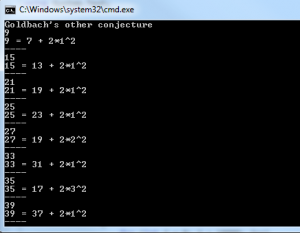# C#: Euler 36

https://projecteuler.net/problem=36

The decimal number, 585 = 10010010012 (binary), is palindromic in both bases.
Find the sum of all numbers, less than one million, which are palindromic in base 10 and base 2.

```//Euler 36 static void Main(string[] args) { int num = 0; int sum = 0; while (num &lt; 1000000) { num += 1; string sBits = Convert.ToString(num, 2); string sNum = num.ToString(); if (isSym(sNum) &amp;&amp; isSym(sBits)) { sum += num; } } Console.WriteLine("Answer : "+sum); }   public static string Reverse(string s) { char[] charArray = s.ToCharArray(); Array.Reverse(charArray); return new string(charArray); }   public static bool isSym(string n) { double half = n.Length / 2; string a = n.ToString(); string b = n.ToString(); b = Reverse(b); string new_a = ""; string new_b = ""; for (int i = 0; i &lt; half; i++) { new_a += a[i]; new_b += b[i]; } if (new_a == new_b) return true; return false; }```

# Visualizing Project Euler Problem 15 using C# Winforms | Lattice Paths

Mapping paths..

```public static Brush aBrush = (Brush)Brushes.Black; public static string path_collect = ""; public static LinkedList<string> complete_paths = new LinkedList<string>(); public bool wall = false; public bool floor = false;   public static int miliseconds = 15;   public int current_x = 10; public int current_y = 10;     public Form1() { InitializeComponent(); }     private void Form1_Load(object sender, EventArgs e) { label3.Text = miliseconds + "ms"; }   private void Form1_Paint(object sender, PaintEventArgs e) { int x = 10; int y = 10; for (int i = 1; i < 21; i++) { for (int j = 1; j < 21; j++) { e.Graphics.DrawEllipse(Pens.Black, x* i , y *j, 6, 6); } } }   private void bntPath_Click(object sender, EventArgs e) { bntPath.Enabled = false; backgroundWorker1.RunWorkerAsync(); }     protected void Move(string path) { Graphics g = this.CreateGraphics();   foreach (char c in path) { if (c == '0') { g.FillRectangle(aBrush, current_x + 10, current_y, 10, 10); current_x += 10; path_collect += "→"; } else if (c == '1') { g.FillRectangle(aBrush, current_x, current_y + 10, 10, 10); current_y += 10; path_collect += "↓"; } }   current_x = 10; current_y = 10;   label2.Text = "New Unique Path\r\n" + path_collect;   Brush NewBrush = new SolidBrush(GetRandomColor()); aBrush = NewBrush; path_collect = "";   }     private void backgroundWorker1_DoWork_1(object sender, DoWorkEventArgs e) { /* while (true) { MoveRandom(); Thread.Sleep(miliseconds); } */ Int64 i = 0; Int64 count_unique_paths = 0;     while (true) { string bits = Convert.ToString(i, 2).PadLeft(38, '0'); string check = bits; check = Regex.Replace(check, "0", ""); if (check.Length == 19) { Move(bits); //Thread.Sleep(50); count_unique_paths += 1; label4.Text = "Distinct Paths " + count_unique_paths; } i += 1; }   }   string row = "";   public bool completerow = true;   private Random random; private Color GetRandomColor() { random = new Random(); return Color.FromArgb(random.Next(0, 255), random.Next(0, 255), random.Next(0, 255)); }   protected void MoveRandom() { Graphics g = this.CreateGraphics(); Random rand = new Random(); int random = rand.Next(0, 2);   if (random == 0) { if (!wall) { g.FillRectangle(aBrush, current_x + 10, current_y, 10, 10); current_x += 10; path_collect += "→"; } } else if (random == 1) { if (!floor) { g.FillRectangle(aBrush, current_x, current_y + 10, 10, 10); current_y += 10; path_collect += "↓"; } } CheckWall(); }   protected void CheckWall() { if (current_x == 200) wall = true; if (current_y == 200) floor = true; if (wall && floor) { current_x = 10; current_y = 10; wall = false; floor = false; if (!complete_paths.Contains(path_collect)) { complete_paths.AddLast(path_collect); label1.Text = "Complete " + complete_paths.Count; label2.Text = "New Unique Path\r\n" + path_collect;   } Brush NewBrush = new SolidBrush(GetRandomColor()); aBrush = NewBrush; path_collect = ""; } }```

//Some fun with random paths at different speeds.